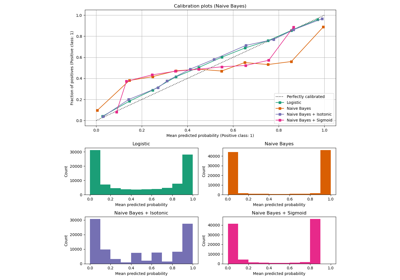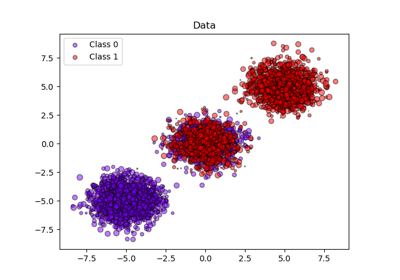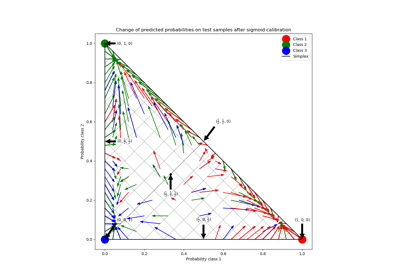# sklearn.calibration.CalibratedClassifierCV¶

class sklearn.calibration.CalibratedClassifierCV(base_estimator=None, *, method='sigmoid', cv=None, n_jobs=None, ensemble=True)[source]

Probability calibration with isotonic regression or logistic regression.

This class uses cross-validation to both estimate the parameters of a classifier and subsequently calibrate a classifier. With default ensemble=True, for each cv split it fits a copy of the base estimator to the training subset, and calibrates it using the testing subset. For prediction, predicted probabilities are averaged across these individual calibrated classifiers. When ensemble=False, cross-validation is used to obtain unbiased predictions, via cross_val_predict, which are then used for calibration. For prediction, the base estimator, trained using all the data, is used. This is the method implemented when probabilities=True for sklearn.svm estimators.

Already fitted classifiers can be calibrated via the parameter cv="prefit". In this case, no cross-validation is used and all provided data is used for calibration. The user has to take care manually that data for model fitting and calibration are disjoint.

The calibration is based on the decision_function method of the base_estimator if it exists, else on predict_proba.

Read more in the User Guide.

Parameters
base_estimatorestimator instance, default=None

The classifier whose output need to be calibrated to provide more accurate predict_proba outputs. The default classifier is a LinearSVC.

method{‘sigmoid’, ‘isotonic’}, default=’sigmoid’

The method to use for calibration. Can be ‘sigmoid’ which corresponds to Platt’s method (i.e. a logistic regression model) or ‘isotonic’ which is a non-parametric approach. It is not advised to use isotonic calibration with too few calibration samples (<<1000) since it tends to overfit.

cvint, cross-validation generator, iterable or “prefit”, default=None

Determines the cross-validation splitting strategy. Possible inputs for cv are:

• None, to use the default 5-fold cross-validation,

• integer, to specify the number of folds.

• An iterable yielding (train, test) splits as arrays of indices.

For integer/None inputs, if y is binary or multiclass, StratifiedKFold is used. If y is neither binary nor multiclass, KFold is used.

Refer to the User Guide for the various cross-validation strategies that can be used here.

If “prefit” is passed, it is assumed that base_estimator has been fitted already and all data is used for calibration.

Changed in version 0.22: cv default value if None changed from 3-fold to 5-fold.

n_jobsint, default=None

Number of jobs to run in parallel. None means 1 unless in a joblib.parallel_backend context. -1 means using all processors.

Base estimator clones are fitted in parallel across cross-validation iterations. Therefore parallelism happens only when cv != "prefit".

See Glossary for more details.

New in version 0.24.

ensemblebool, default=True

Determines how the calibrator is fitted when cv is not 'prefit'. Ignored if cv='prefit'.

If True, the base_estimator is fitted using training data and calibrated using testing data, for each cv fold. The final estimator is an ensemble of n_cv fitted classifer and calibrator pairs, where n_cv is the number of cross-validation folds. The output is the average predicted probabilities of all pairs.

If False, cv is used to compute unbiased predictions, via cross_val_predict, which are then used for calibration. At prediction time, the classifier used is the base_estimator trained on all the data. Note that this method is also internally implemented in sklearn.svm estimators with the probabilities=True parameter.

New in version 0.24.

Attributes
classes_ndarray of shape (n_classes,)

The class labels.

n_features_in_int

Number of features seen during fit. Only defined if the underlying base_estimator exposes such an attribute when fit.

New in version 0.24.

calibrated_classifiers_list (len() equal to cv or 1 if cv="prefit" or ensemble=False)

The list of classifier and calibrator pairs.

• When cv="prefit", the fitted base_estimator and fitted calibrator.

• When cv is not “prefit” and ensemble=True, n_cv fitted base_estimator and calibrator pairs. n_cv is the number of cross-validation folds.

• When cv is not “prefit” and ensemble=False, the base_estimator, fitted on all the data, and fitted calibrator.

Changed in version 0.24: Single calibrated classifier case when ensemble=False.

References

1

Obtaining calibrated probability estimates from decision trees and naive Bayesian classifiers, B. Zadrozny & C. Elkan, ICML 2001

2

Transforming Classifier Scores into Accurate Multiclass Probability Estimates, B. Zadrozny & C. Elkan, (KDD 2002)

3

Probabilistic Outputs for Support Vector Machines and Comparisons to Regularized Likelihood Methods, J. Platt, (1999)

4

Predicting Good Probabilities with Supervised Learning, A. Niculescu-Mizil & R. Caruana, ICML 2005

Examples

>>> from sklearn.datasets import make_classification
>>> from sklearn.naive_bayes import GaussianNB
>>> from sklearn.calibration import CalibratedClassifierCV
>>> X, y = make_classification(n_samples=100, n_features=2,
...                            n_redundant=0, random_state=42)
>>> base_clf = GaussianNB()
>>> calibrated_clf = CalibratedClassifierCV(base_estimator=base_clf, cv=3)
>>> calibrated_clf.fit(X, y)
CalibratedClassifierCV(base_estimator=GaussianNB(), cv=3)
>>> len(calibrated_clf.calibrated_classifiers_)
3
>>> calibrated_clf.predict_proba(X)[:5, :]
array([[0.110..., 0.889...],
[0.072..., 0.927...],
[0.928..., 0.071...],
[0.928..., 0.071...],
[0.071..., 0.928...]])

>>> from sklearn.model_selection import train_test_split
>>> X, y = make_classification(n_samples=100, n_features=2,
...                            n_redundant=0, random_state=42)
>>> X_train, X_calib, y_train, y_calib = train_test_split(
...        X, y, random_state=42
... )
>>> base_clf = GaussianNB()
>>> base_clf.fit(X_train, y_train)
GaussianNB()
>>> calibrated_clf = CalibratedClassifierCV(
...     base_estimator=base_clf,
...     cv="prefit"
... )
>>> calibrated_clf.fit(X_calib, y_calib)
CalibratedClassifierCV(base_estimator=GaussianNB(), cv='prefit')
>>> len(calibrated_clf.calibrated_classifiers_)
1
>>> calibrated_clf.predict_proba([[-0.5, 0.5]])
array([[0.936..., 0.063...]])


Methods

 fit(X, y[, sample_weight]) Fit the calibrated model. get_params([deep]) Get parameters for this estimator. Predict the target of new samples. Calibrated probabilities of classification. score(X, y[, sample_weight]) Return the mean accuracy on the given test data and labels. set_params(**params) Set the parameters of this estimator.
fit(X, y, sample_weight=None)[source]

Fit the calibrated model.

Parameters
Xarray-like of shape (n_samples, n_features)

Training data.

yarray-like of shape (n_samples,)

Target values.

sample_weightarray-like of shape (n_samples,), default=None

Sample weights. If None, then samples are equally weighted.

Returns
selfobject

Returns an instance of self.

get_params(deep=True)[source]

Get parameters for this estimator.

Parameters
deepbool, default=True

If True, will return the parameters for this estimator and contained subobjects that are estimators.

Returns
paramsdict

Parameter names mapped to their values.

predict(X)[source]

Predict the target of new samples. The predicted class is the class that has the highest probability, and can thus be different from the prediction of the uncalibrated classifier.

Parameters
XThe samples, as accepted by base_estimator.predict
Returns
Cndarray of shape (n_samples,)

The predicted class.

predict_proba(X)[source]

Calibrated probabilities of classification.

This function returns calibrated probabilities of classification according to each class on an array of test vectors X.

Parameters
XThe samples, as accepted by base_estimator.predict_proba
Returns
Cndarray of shape (n_samples, n_classes)

The predicted probas.

score(X, y, sample_weight=None)[source]

Return the mean accuracy on the given test data and labels.

In multi-label classification, this is the subset accuracy which is a harsh metric since you require for each sample that each label set be correctly predicted.

Parameters
Xarray-like of shape (n_samples, n_features)

Test samples.

yarray-like of shape (n_samples,) or (n_samples, n_outputs)

True labels for X.

sample_weightarray-like of shape (n_samples,), default=None

Sample weights.

Returns
scorefloat

Mean accuracy of self.predict(X) wrt. y.

set_params(**params)[source]

Set the parameters of this estimator.

The method works on simple estimators as well as on nested objects (such as Pipeline). The latter have parameters of the form <component>__<parameter> so that it’s possible to update each component of a nested object.

Parameters
**paramsdict

Estimator parameters.

Returns
selfestimator instance

Estimator instance.

## Examples using sklearn.calibration.CalibratedClassifierCV¶Probability Calibration curves¶Probability calibration of classifiers¶Probability Calibration for 3-class classification¶